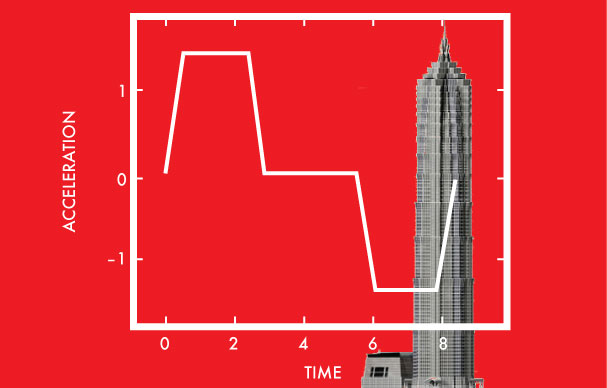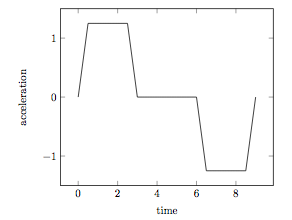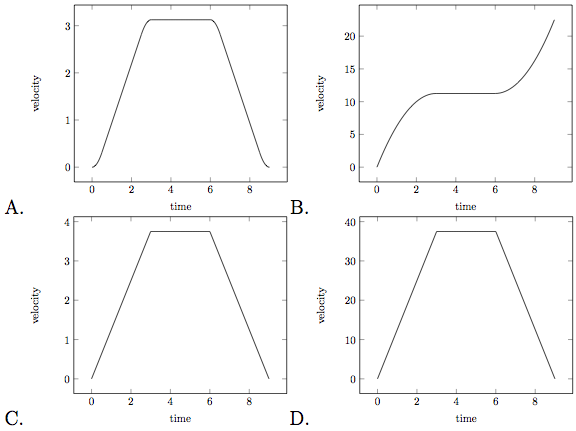Calculus

# Displacement, Velocity, Acceleration (Integrals): Level 2-3 Challenges

A point P is moving on a number line, and after $t$ seconds its velocity, $v$ cm/sec, is $v = 4t-12$. Find the actual distance traveled by point P from $t = 0$ to $t = 8$.The Shanghai Tower has an architectural height of 632 meters, and is equipped with the world's fastest elevator which can travel at $18 m/s$. If we assume that it travels at its maximum speed, then it would take just under 36 seconds to reach the top. However, this assumption is unrealistic, as the elevator needs time to increase its velocity, i.e. accelerate.

In order to travel up several stories, the acceleration of the elevator has the following shape:Which of the following is the best graph which illustrates the velocity of the elevator?Two cars, 270 miles apart, start driving toward each other. One car has a speed of 40mph and the other has a speed of 50mph. Chris stands at the midpoint between the two cars. He runs toward one of the cars, touches its hood, then runs towards the other car, touches its hood, and repeats this until the cars collide (and presumably bounce off of him). Chris travels at a speed of 60mph at all times.

Let $v(t)$ denote Chris’s velocity in mph and $t_0$ denote the amount of time that has elapsed at the moment the cars collide (and presumably bounce off of Chris). Evaluate:

$\left|\int_{0}^{t_0}v(t)dt\right| + \int_{0}^{t_0}\left|v(t)\right|dt$

A body starts from rest and travels a distance $S$ with uniform acceleration, then moves a distance $2S$ uniformly, and then finally comes to rest after moving further $5S$ under uniform deceleration.

What is the ratio of the body's average velocity to maximum velocity?

The acceleration of a particle traveling in a straight line is given by the equation $a(t)=2t.$ If the velocity at $t=1$ is $v=6$ and the distance from the starting point at $t=1$ is $s=17$, what is the distance from the starting point at $t=2?$

×